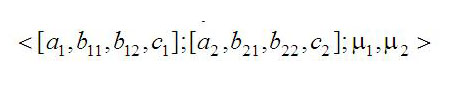DOI: https://doi.org/10.15802/stp2013/11375

### Reliability of fuzzy systems evaluation methodology using different types of vague sets

A. A. Kosolapov

#### Abstract

Purpose. Development of the reliability calculating methodology of fuzzy systems with components, reliability parameters of which are described by the membership functions of various kinds. Methodology. Systematic analysis of the known approaches to the assessment of the reliability of fuzzy systems with vague boundaries and different kinds of membership functions. Findings. A unified description and the new tabular algorithm of various arithmetic operations run between the various types of fuzzy sets are proposed and tabular methods of reliability analysis of the basic structures of fuzzy systems are developed. Originality. A generalized description of triangular and trapezoid-shaped accessory functions of fuzzy boundaries in a special tuples is introduced, analytical expressions for the formation of tabular schedules of their functions and the calculation of the reliability of fuzzy systems with complex structures are obtained: sequential, redundant - parallel, serial-parallel, parallel-serial. The practical value.The obtained models and tabular algorithms simplify the calculations of the reliability of complex electro-mechanical human-machine automation systems with fuzzy parameters and vague boundaries.

#### Keywords

fuzzy reliability; fuzzy sets; vague sets; membership function of true (MFT); membership function of lies (MFL); table models

PDF (Русский)

#### References

Kosolapov A.A. Metodika analiza nadezhnosti nechetkikh sistem s ispolzovaniyem teorii razmytykh mnozhestv [The method of fuzzy systems reliability analysis using fuzzy set theory]. Materialy mezhdu-narodnoy nauchno-prakticheskoy konferentsii “Sovremennyye napravleniya teoreticheskikh i prikladnykh issledovaniy ‘2013” [Proc. of Int. Theoretical and Practical Conf. “Modern directions of theoretical and ap-plied research '2013”]. Odessa, 2013, pp. 71-81.

Cai K.Y., Wen C.Y., Zhang M.L. Possibistic reliability behavior of typical systems with two types of failures. Fuzzy Sets and Systems, 1991, no. 43, 1, pp. 17-32.

Chen S.M. Analyzing fuzzy system reliability using interval valued vague set theory. International Journal of Applied Science and Engineering, 2003, no. l, 1, pp. 82-88.

Cheng C.H., Mon D.L. Fuzzy system reliability analysis by interval of confidence. Fuzzy Sets and Systems, 1993, no. 56, 1, pp. 29-35.

Gau W.L., Buehrer D.J. Interval valued vague sets. IEEE. Transactions on Systems Maa and Cybernetics, 1993, no. 23, 2. pp. 610- 614.

Kumar A., Yadav S.P., Kumar S. Fuzzy System Reliability Using Different Types of Vague Sets. Int. Journal of Applied Science and Engineering, 2008, no. 6, 1, pp. 71-83.

Mon D.L., Cheng C.H. Fuzzy system reliability analysis for components with different membership func-tions. Fuzzy Sets and Systems, 1994, no. 64, 2, pp. 145-157.

Singer D.A. Fuzzy set approach to fault tree and reliability analysis. Fuzzy Sets and Systems, 1990, no. 34, 2, pp. 145-155.

Chang J.R., Chang K.H., Liao S.H., Cheng C.H. The reliability of general vague fault-tree analysis of weap-on systems fault diagnosis. vSoft Computing, 2006, no. 10, pp. 531-542.

Zadeh L.A. Fuzzy sets. Inform. Control, 1965, no. 8, 3, pp. 338-353.

#### GOST Style Citations

1. Косолапов, А. А. Методика анализа надёжности нечётких систем с использованием теории размытых множеств / А. А. Косолапов // Современные направления теоретических и прикладных исследований 2013 : мат. междунар. науч.-практ. конф. – Одесса : КУПРИЕНКО, 2013. – С. 71–81.

2. Cai, K. Y. Possibistic reliability behavior of typical systems with two types of failures / K. Y. Cai, C. Y. Wen, M. L. Zhang // Fuzzy Sets and Systems. – 1991. − № 43, 1. – P. 17–32.

3. Chen, S. M. Analyzing fuzzy system reliability using interval valued vague set theory // International Journal of Applied Science and Engineering. − 2003. – № l, 1. − P. 82−88.

4. Cheng, C. H. Fuzzy system reliability analysis by interval of confidence / C. H. Cheng, D. L. Mon // Fuzzy Sets and Systems. − 1993. − № 56, 1. − P. 29−35.

5. Gau, W. L. Interval valued vague sets / W. L. Gau, D. J. Buehrer // IEEE. Transactions on Systems Maa and Cybernetics. − 1993. − № 23, 2. − P. 610−614.

6. Kumar, A. Fuzzy System Reliability Using Different Types of Vague Sets / A. Kumar, S. P. Yadav, S. Kumar // Int. Journal of Applied Science and Engineering. − 2008. − № 6, 1. − P. 71−83.

7. Mon, D. L. Fuzzy system reliability analysis for components with different membership functions / D. L. Mon, C. H. Cheng // Fuzzy Sets and Systems. − 1994. − № 64, 2. − P. 145−157.

8. Singer, D. A. Fuzzy set approach to fault tree and reliability analysis / D. A. Singer // Fuzzy Sets and Systems. − 1990. − № 34, 2. − P. 145−55.

9. The reliability of general vague fault-tree analysis of weapon systems fault diagnosis / Chang J. R., Chang K. H., Liao S. H., Cheng C. H. // Soft Computting. − 2006. − № 10. − P. 531−542.

10. Zadeh, L. A. Fuzzy sets / L. A. Zaden // Inform. Control. − 1965. − № 8, 3. − P. 338−353.